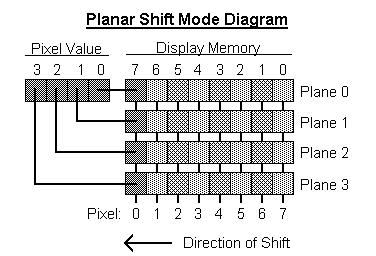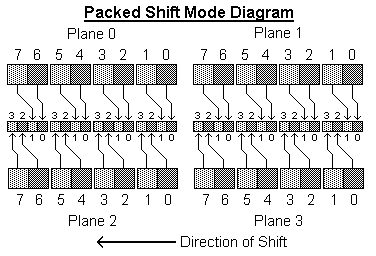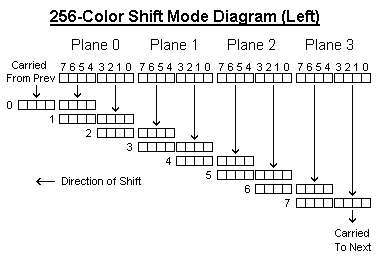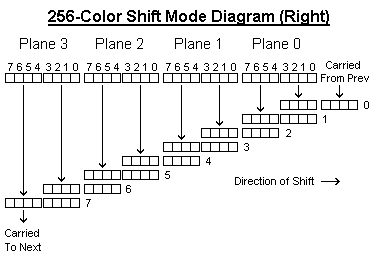Home Back
Hardware Level VGA and SVGA Video Programming Information Page
VGA Sequencer Operation
Introduction
The sequencer portion of the VGA hardware reads the display memory and converts it into data that is sent to the attribute controller.  This would normally be a simple part of the video hardware, but the VGA hardware was designed to provide a degree of software compatibility with monochrome, CGA, EGA, and MCGA adapters.  For this reason, the sequencer has quite a few different modes of operation.  Further complicating programming, the sequencer has been poorly documented, resulting in many variances between various VGA/SVGA implementations.

The sequencer operates by loading a display address into memory, then shifting it out pixel by pixel.   The memory is organized internally as 64K addresses, 32 bits wide.  The seqencer maintains an internal 16-bit counter that is used to calculate the actual index of the 32-bit location to be loaded and shifted out.  There are several different mappings from this counter to actual memory addressing, some of which use other bits from other counters, as required to provide compatibility with older hardware that uses those addressing schemes.

<More to be added here>

Graphics Shifting Modes
When the Alphanumeric Mode Disable field is set to 1, the sequencer operates in graphics mode where data in memory references pixel values, as opposed to the character map based operation used for alphanumeric mode.
The sequencer has three methods of taking the 32-bit memory location loaded and shifting it into 4-bit pixel values suitable for graphics modes, one of which combines 2 pixel values to form 8-bit pixel values.  The first method is the one used for the VGA's 16 color modes.  This mode is selected when both the 256-Color Shift Mode and Shift Register Interleave Mode fields are set to 0.  In this mode, one bit from each of the four 8-bit planes in the 32-bit memory is used to form a 16 color value. This is shown in the diagram below, where the most significant bit of each of the four planes is shifted out into a pixel value, which is then sent to the attribute controller to be converted into an index into the DAC palette.  Following this, the remaining bits will be shifted out one bit at a time, from most to least significant bit, with the bits from planes 0-3 going to pixel bits 0-3.The second shift mode is the packed shift mode, which is selected when both the 256-Color Shift Mode field is set to 0 and the Shift Register Interleave Mode field is set to 1.This is used by the VGA bios to support video modes compatible with CGA video modes.  However, the CGA only uses planes 0 and 1 providing for a 4 color packed mode; however, the VGA hardware actually uses bits from two different bit planes, providing for 16 color modes.  The bits for the first four pixels shifted out for a given address are stored in planes 0 and 2.  The second four are stored in planes 1 and 3.  For each pixel, bits 3-2 are shifted out of the higher numbered plane and bits 1-0 are shifted out of the lower numbered plane.  For example, bits 3-2 of the first pixel shifted out are located in bits 7-6 of plane 2; likewise, bits 1-0 of the same pixel are located in bits 7-6 of plane 0.The third shift mode is used for 256-color modes, which is selected when the 256-Color Shift Mode field is set to 1 (this field takes precedence over the Shift Register Interleave Mode field.)  This behavior of this shift mode varies among VGA implementations, due to it normally being used in combination with the 8-bit Color Enable field of the attribute controller.  Thus certain variances in the sequencing operations can be masked by similar variances in the attribute controller.  However, the implementations I have experimented with seem to fall into one of two similar behaviors, and thus it is possible to describe both here.  Note that one is essentially a mirror image of the other, leading me to believe that the designers knew how it should work to be 100% IBM VGA compatible, but managed to get it backwards in the actual implementation. Due to being very poorly documented and understood, it is very possible that there are other implementations that vary significantly from these two cases.  I do, however, feel that attempting to specify each field's function as accurately possible can allow more powerful utilization of the hardware.
When this shift mode is enabled, the VGA hardware shifts 4 bit pixel values out of the 32-bit memory location each dot clock.  This 4-bit value is processed by the attribute controller, and the lower 4 bits of the resulting DAC index is combined with the lower 4 bits of the previous attribute lookup to produce an 8-bit index into the DAC palette.  This is why, for example, a 320 pixel wide 256 color mode needs to be programmed with timing values for a 640 pixel wide normal mode.  In 256-color mode, each plane holds a 8-bit value which is intended to be the DAC palette index for that pixel.  Every second 8-bit index generated should correspond to the values in planes 0-3, appearing left to right on the display.  This is masked by the attribute controller, which in 256 color mode latches every second 8-bit value as well.  This means that the intermediate 8-bit values are not normally seen, and is where implementations can vary.  Another variance is whether the even or odd pixel values generated are the intended data bytes.  This also is masked by the attribute controller, which latches the appropriate even or odd pixel values.
The first case is where the 8-bit values are formed by shifting the 4 8-bit planes left.  This is shown in the diagram below.  The first pixel value generated will be the value held in bits 7-4 of plane 0, which is then followed by bits 3-0 of plane 0.  This continues, shifting out the upper four bits of each plane in sequence before the lower four bits, ending up with bits 3-0 of plane 3.  Each pixel value is fed to the attribute controller, where a lookup operation is performed using the attribute table.  The previous 8-bit DAC index is shifted left by four, moving from the lower four bits to the upper four bits of the DAC index, and the lower 4 bits of the attribute table entry for the current pixel is shifted into the lower 4 bits of the 8-bit value, producing a new 8-bit DAC index.  Note how one 4-bit result carries over into the next display memory location sequenced.
For example, assume planes 0-3 hold 01h, 23h, 45h, and 67h respectively, and the lower 4 bits of the the attribute table entries hold the value of the index itself, essentially using the index value as the result, and the last 8-bit DAC index generated was FEh. The first cycle, the pixel value generated is 0h, which is fed to the attribute controller and looked up, producing the table entry 0h (surprise!) The previous DAC index, FEh, is shifted left by four bits, while the new value, 0h is shifted into the lower four bits.  Thus, the new DAC index output for this pixel is E0h.  The next pixel is 1h, which produces 1h at the other end of the attribute controller.  The previous DAC index, E0h is shifted again producing 01h.  This process continues, producing the DAC indexes, in order, 12h, 23h, 34h, 45h, 56h, and 67h.  Note that every second DAC index is the appropriate 8-bit value for a 256-color mode, while the values in between contain four bits of the previous and four bits of the next DAC index.The second case is where the 8-bit values are formed by shifting the 8-bit values right, as depicted in the diagram below.  The first pixel value generated is the lower four bits of plane 0, followed by the upper four bits.  This continues for planes 1-3 until the last pixel value produced, which is the upper four bits of Plane 3.  These pixel values are fed to the attribute controller, where the corresponding entry in the attribute table is looked up.  The previous 8-bit DAC index is shifted right 4 places. and the lower four bits of the attribute table entry generated is used as the upper four bits of the new DAC index.
For example, assume planes 0-3 hold 01h, 23h, 45h, and 67h respectively, and the lower 4 bits of the the attribute table entries hold the value of the index itself, essentially using the index value as the result, and the last 8-bit DAC index generated was FEh. The first cycle, the pixel value generated is 1h, which is fed to the attribute controller and looked up, producing the table entry 1h. The previous DAC index, FEh, is shifted right by four bits, while the new value, 1h is shifted into the upper four bits.  Thus, the new DAC index output for this pixel is 1Fh.  The next pixel is 0h, which produces 0h at the other end of the attribute controller.  The previous DAC index, 1Fh is shifted again producing 01h.  This process continues, producing the DAC indexes, in order, 30h, 23h, 52h, 45h, 74h, and 67h.  Again, note that every second DAC index is the appropriate 8-bit value for a 256-color mode, while the values in between contain four bits of the previous and four bits of the next DAC index.Another variance that can exist is whether the first or second DAC index generated at the beginning of a scan line is the appropriate 8-bit value.  If it is the second, the first DAC index contains 4 bits from the contents of the DAC index prior to the start of the scan line.  This could conceivably contain any value, as it is normally masked by the attribute controller when in 256-color mode whcih would latch the odd pixel values.  Likely this value will be either 00h or whatever the contents were at the end of the previous scan line.  A similar circumstance arises where the last pixel value generated falls on a boundary between memory addresses.  In this circumstance, however, the value generated is produced by sequencing the next display memory address as if the line continued, and is thus more predictable.

Notice: All trademarks used or referred to on this page are the property of their respective owners.
All pages are Copyright © 1997, 1998, J. D. Neal, except where noted. Permission for utilization and distribution is subject to the terms of the FreeVGA Project Copyright License.